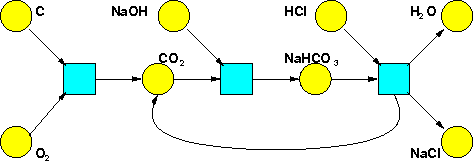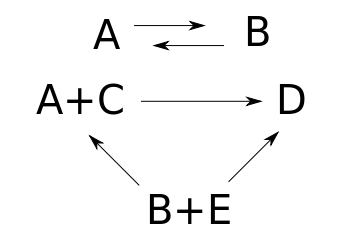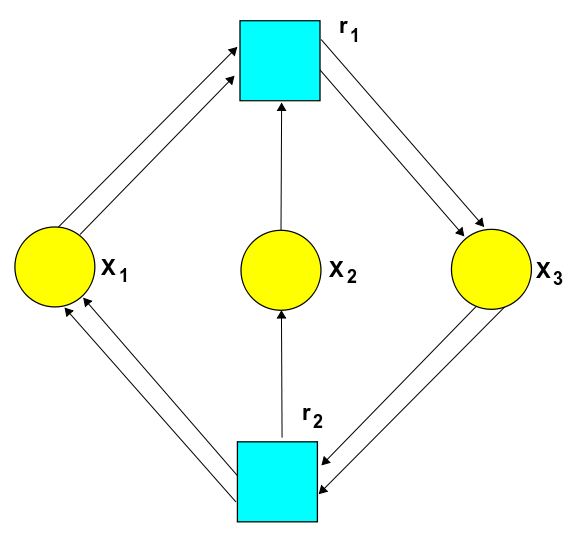# The Azimuth Project Blog - network theory (part 17)

This page is a blog article in progress, written by Jacob Biamonte and John Baez. To see discussions of it as it was being written, go to the Azimuth Forum. For the final polished version, visit the Azimuth Blog.

joint with Jacob Biamonte

We’ve seen how Petri nets can be used to describe chemical reactions. Indeed our very first example came from chemistry:However, chemists rarely use the formalism of Petri nets. They use a different but entirely equivalent formalism, called ‘reaction networks’. So now we’d like to tell you about those.

You may wonder: why bother with another formalism, if it’s equivalent to the one we’ve already seen? Well, one goal of this network theory program is to get people from different subjects to talk to each other—or at least be able to. This requires setting up some dictionaries to translate between formalisms. Furthermore, lots of deep results on stochastic Petri nets are being proved by chemists—but phrased in terms of reaction networks. So you need to learn this other formalism to read their papers. Finally, this other formalism is actually better in some ways!

Reaction networks

Here’s a reaction network:This network involves 5 species: that is, different kinds of things. They could be atoms, molecules, ions or whatever: chemists call all of these species, and there’s no need to limit the applications to chemistry: in population biology, they could even be biological species! We’re calling them A, B, C, D, and E, but in applications, we’d call them by specific names like CO2 and HCO3-, or ‘rabbit’ and ‘wolf’, or whatever.

This network also involves 5 reactions, which are shown as arrows. Each reaction turns one bunch of species into another. So, written out more longwindedly, we’ve got these reactions:

A $\to$ B

B $\to$ A

A + C $\to$ D

B + E $\to$ A + C

B + E $\to$ D

If you remember how Petri nets work, you can see how to translate any reaction network into a Petri net, or vice versa. For example, the reaction network we’ve just seen gives this Petri net:Each species corresponds to a state of this Petri net, drawn as a yellow circle. And each reaction corresponds to transition of this Petri net, drawn as a blue square. The arrows say how many things of each species appear as input or output to each transition. There’s less explicit emphasis on complexes in the Petri net notation, but you can read them off if you want them.

In chemistry, a bunch of species is called a ‘complex’. But what do I mean by ‘bunch’, exactly? Well, I mean that in a given complex, each species can show up 0,1,2,3… or any natural number of times. So, we can formalize things like this:

Definition. Given a set $S$ of species, a complex of those species is a function $C: S \to \mathbb{N}$.

Roughly speaking, a reaction network is a graph whose vertices are labelled by complexes. Unfortunately, the word ‘graph’ means different things in mathematics—appallingly many things! Everyone agrees that a graph has vertices and edges, but there are lots of choices about the details. Most notably:

• We can either put arrows on the edges, or not.

• We can either allow more than one edge between vertices, or not.

• We can either allow edges from a vertex to itself, or not.

If we say ‘no’ in every case we get the concept of ‘simple graph’, which we discussed last time. At the other extreme, if we say ‘yes’ in every case we get the concept of ‘directed multigraph’, which is what we want now. A bit more formally:

Definition. A directed multigraph consists of a set $V$ of vertices, a set $E$ of edges, and functions $s,t: E \to V$ saying the source and target of each edge.

Given this, we can say:

Definition. A reaction network is a set of species together with a directed multigraph whose vertices are labelled by complexes of those species.

You can now prove that reaction networks are equivalent to Petri nets:

Puzzle 1. Show that any reaction network gives a Petri net, and vice versa.

In a stochastic Petri net each transition is labelled by a rate constant: that is, a numbers in $[0,\infty)$. This lets us write down some differential equations saying how species turn into each other. So, let’s make this definition (which is not quite standard):

Definition. A stochastic reaction network is a reaction network where each reaction is labelled by a rate constant.

Now you can do this:

Puzzle 2. Show that any stochastic reaction network gives a stochastic Petri net, and vice versa.

For extra credit, show that in each of these puzzles we actually get an equivalence of categories! For this you need to define morphisms between Petri nets, morphisms between reaction networks, and similarly for stochastic Petric nets and stochastic reaction networks. If you get stuck, ask Eugene Lerman for advice. There are different ways to define morphisms, but he knows a cool one.

We’ve been downplaying category theory so far, but it’s been lurking beneath everything we do, and someday it may rise to the surface.

#### The deficiency zero theorem

You may have already noticed one advantage of reaction networks over Petri nets: they’re quicker to draw. This is true even for tiny examples. For example, this reaction network:

$2 X_1 + X_2 \leftrightarrow 2 X_3$

corresponds to this Petri net:But there’s also a deeper advantage. As we saw in Part 8, any stochastic Petri net gives two equations:

• The master equation, which says how the probability that we have a given number of things of each species changes with time.

• The rate equation, which says how the expected number of things in each state changes with time.

The simplest solutions of these equations are the equilibrium solutions, where nothing depends on time. Back in Part 9, we explained when an equilibrium solution of the rate equation gives an equilibrium solution of the master equation. But when is there an equilibrium solution of the rate equation in the first place?

Feinberg’s ‘deficiency zero theorem’ gives a handy sufficient condition. And this condition is best stated using reaction networks! But to understand it, we need to understand the ‘deficiency’ of a reaction network. So let’s define that, and they say what all the words in the definition mean:

Definition. The deficiency of a reaction complex is:

• the number of vertices

minus

• the number of connected components

minus

• the dimension of the stochiometric subspace.

The first two concepts here are easy. A reaction network is a graph (okay, a directed multigraph). So, it has some number of vertices, and also some number of connected components. Two vertices lie in the same connected component iff you can get from one to the other by a path where you don’t care which way the arrows point. For example, this reaction network:has 5 vertices and 2 connected components.

So, what’s the ‘stochiometric subspace’? ‘Stochiometry’ is a scary-sounding word, but it’s basically just the art of counting species. To do this, we can form a vector space $\mathbb{R}^S$ where $S$ is the set of species. A vector in $\mathbb{R}^S$ is a function from species to real numbers, saying how much of each species is present. Any complex gives a vector in $\mathbb{R}^S$, because it’s actually a function from species to natural numbers.

Definition. The stochiometric subspace of a reaction network is the subspace $\mathrm{Stoch} \subseteq \mathbb{R}^S$ spanned by vectors of the form $x - y$ where $x$ and $y$ are complexes connected by a reaction.

This makes sense because vertices in the reaction network are complexes, and edges are reactions. Let’s see how it works in our example:Each complex here can be seen as a vector in $\mathbb{R}^S$, which is a vector space with basis A, B, C, D, E. Each reaction gives a difference of two vectors coming from complexes:

• The reaction A $\to$ B gives the vector B - A.

• The reaction B $\to$ A gives the vector A - B.

• The reaction A + C $\to$ D gives the vector D - A - C.

• The reaction B + E $\to$ A + C gives the vector A + C - B - E.

• The reaction B + E $\to$ D gives the vector D - B - E.

The pattern is obvious, I hope.

These 5 vectors span the stochiometric subspace. But this subspace isn’t 5-dimensional, because these vectors are linearly dependent! The first vector is the negative of the second one. The last is the sum of the previous two. And those are all the linear dependencies, so the stochiometric subspace is 3 dimensional. For example, it’s spanned by these 3 linearly independent vectors: A - B, D - A - C, and D - B - E.

I hope you see the moral of this example: the stochiometric subspace is the space of ways to move in $\mathbb{R}^S$ that are allowed by the reactions in our reaction network! And this is important because the rate equation describes how the amount of each species changes as time passes. So, it describes a point moving around in $\mathbb{R}^S$.

Thus, if $\mathrm{Stoch} \subseteq \mathbb{R}^S$ is the stochiometric subspace, and $x(t) \in \mathbb{R}^S$ is a solution of the rate equation, then $x(t)$ always stays within the set

$x(0) + \mathrm{Stoch} = \{ x(0) + y \colon \; y \in \mathrm{Stoch} \}$

Mathematicians would call this set the coset of $x(0)$, but chemists call it the <b>stochiometric compatibility class</i> of $x(0)$.

Anyway: what’s the deficiency of the reaction complex in our example? It’s

$5 - 2 - 3 = 0$

since there are 5 complexes, 2 connected components and the dimension of the stochiometric subspace is 3.

But what’s the deficiency zero theorem? You’re almost ready for it. You just need to know one more piece of jargon! A reaction network is weakly reversible if whenever there’s a reaction going from a complex $x$ to a complex $y$, there’s a path of reactions going back from $y$ to $x$. Here the paths need to follow the arrows.

So, this reaction network is not weakly reversible:since we can get from A + C to D but not back from D to A + C. However, if we added just two reverse reactions to the triangle, it would be.

If a reaction network isn’t weakly reversible, one complex can gradually turn into another, but not vice versa. In this situation, what typically happens is that as $t \to +\infty$ we’ll have less and less of one species. We could have an equilibrium where there’s none of this species. But we have little right to expect an equilibrium solution of the rate equation that’s positive, meaning that it sits at a point $x \in (0,infty)^S$, where there’s a nonzero amount of every species.

My argument here is not watertight, but it can be made so when our reaction network has deficiency zero:

Deficiency Zero Theorem (Feinberg). Suppose we are given a reaction network with a finite set of species $S$, and suppose its deficiency is zero. Then:

(i) If the network is not weakly reversible and the rate constants are positive, the rate equation does not have a positive equilibrium solution.

(ii) If the network is not weakly reversible and the rate constants are positive, the rate equation does not have a positive periodic solution, that is, a periodic solution lying in $(0,infty)^S$.

(iii) If the network is weakly reversible and the rate constants are positive, the rate equation has exactly one equilibrium solution in each positive stoichiometric compatibility class.
Any sufficiently nearby solution that starts in the same stochiometric compatibility class will approach this equilibrium as $t \to +\infty$. Furthermore, there are no other positive periodic solutions.

This is quite an impressive result. We’ll do an example or two next time.

#### References and remarks

The deficiency zero theorem was published here:

These other explanations are also very helpful:

At first glance the deficiency zero theorem might seem to settle all the basic questions about the dynamics of reaction networks, or stochastic Petri nets… but actually, it means that deficiency zero reaction networks don’t display very interesting dynamics in the limit as $t \to +\infty$. So, to get more interesting behavior, we need to look at reaction networks that don’t have deficiency zero.

For example, in biology it’s interesting to think about ‘bistable’ chemical reactions: reactions that have two stable equilibria. An electrical switch of the usual sort is a bistable system: it has stable ‘on’ and ‘off’ positions. A bistable chemical reaction can serve as a kind of biological switch:

It’s also interesting to think about chemical reactions with stable periodic solutions. Such a reaction can serve as a biological clock:

category: blog